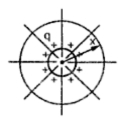### Electric Field of a Long Straight Conductor

Electric potential at a point due to a charge is the amount of work done in bringing a unit positive charge from infinity to that point. The concept of electrical potential is important for the evaluation of the capacitance.
While considering the inductance, magnetic field is important. Similarly while working with capacitance, electric field plays important role. Lines of electric flux originate on the positive charges of one conductor and terminate on the negative charges of other conductor. The total electric flux emanating from a conductor is numerically equal to the coulamb of charge on the conductor. Electric flux density is the electric flux per square meter and is measured in coulamb per square meter.
Consider a long, straight cylindrical conductor placed in uniform medium such as air as shown in the Fig. 1. It has uniform charge along its entire length. It is isolated from other charges so that the charge is uniformly distributed around the periphery. The flux lines are radial.Fig. 1

The electric flux density at x meters from the conductor can be obtained by assuming a cylindrical surface concentric with the conductor and x meters in radius. As all the parts of the surface are at the same distance from the conductor having uniformly distributed charge, the cylindrical surface is a surface of equipotential and the electric flux density on the surface is equal to the flux leaving the conductor per meter of length divided by the line area of the surface in an axial length of 1 m.
Here q is the charge on the conductor and x is the distance from the conductor to the point where it is required to find field intensity. If the filed density is divided by the permittivity of the medium then we get the field intensity.
Permittivity,         ε = εo εr   where
εo = Permittivity of free space = 8.85 x 10-12   F/m
εr   = Relative permittivity
As x approaches infinity, the value of E approaches zero. Therefore the potential difference between conductor and neutral plane at infinity distance where E and potential is zero, is given by,

1.As a person you should know what are your priorities and goals so that it is easier for you to determine and decide when is the time to give up or go on.
Thanks for sharing such a wonderful article, I hope you could inspire more people. Visit my site for an offer you wouldn't want to refuse.

jovie

www.n8fan.net

2.Thank you for posting some kind of information. It was really helpful since I am doing some research now.

Say
www.imarksweb.org

3.Multimeters are often used as hand-held tools for doing basic troubleshooting and field service measurements. Multimeter

4.The writer has written this blog in the most artistic way. Splendid!
visit here